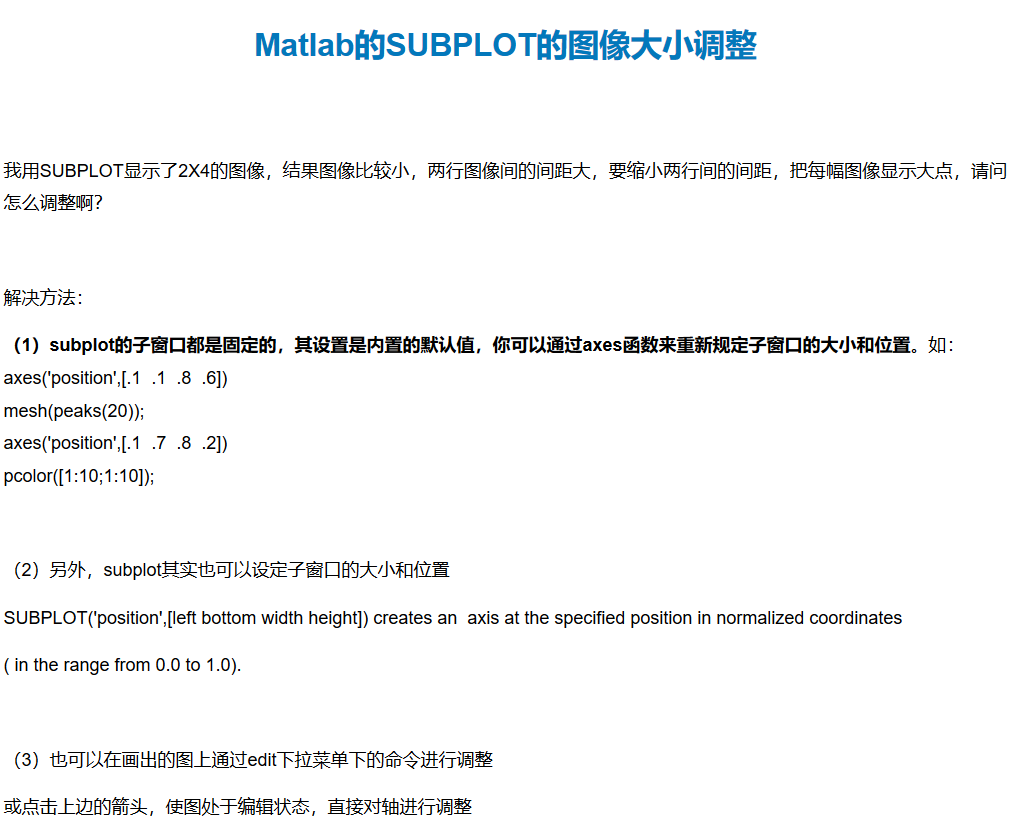•matlab
• 按给定轴移动饼图，和/或调整图的大小。 这允许在同一图上可视化多个饼图，或将饼图与其他图集成。 基于 Walter Robinson 的解决方案。 原始线程可以在这里找到： ...matlab
• 写论文时，图表线条及坐标轴的修饰是很麻烦的，本程序可以解决常用的修饰问题。最后保存图片。
• 在图像处理过程中，经常会对图片的大小进行修改，传统的人工修改的速度较慢，这里利用MATLAB实现对多幅图像大小的修改，并且可以自定义所要修改图片的输出大小
• Matlab 两种比较三个数的大小

千次阅读 2019-12-03 11:11:47
J1 = 0.1; J2 = 0.2; J3 = 0.05; if (J1 <= J2 && J1 <= J3) % min = J1; J = 1; else if (J2 <= J3) % min = J2; J = 2; else % min = J3; J = 3; ...
J1 = 0.1;
J2 = 0.2;
J3 = 0.05;
if (J1 <= J2 && J1 <= J3)
%     min = J1;
J = 1;
else if (J2 <= J3)
%         min = J2;
J = 2;
else
%         min = J3;
J = 3;
end
end
J
%另一种更简洁的方法
a = [J1 J2 J3];
[m index] = min(a);
J = index

展开全文代码
• 绘制流函数的等高线，根据当地速度的大小着色。 与 STREAMCOLOR 类似，除了这里可以直接确保行在流函数空间中均匀分布。matlab
• 读取excel表格数据，包含x,y,z三列数据，本文件能绘制出x数据为横轴，y数据为纵轴，z数据用颜色深浅来表示大小，当固定x或y，能直观的看出z与y或x的关系，在科技论文写作里绘制这样的图，可以给论文加分
• MATLAB数组元素的排序

千次阅读 2021-04-24 00:44:37
MATLAB sort() 函数用来对数组元素进行排序，它有以下几种常见的用法：B = sort(A) %使用默认规则对数组排序B = sort(A, dim) %dim指定排序的维度B = sort(___, direction) %direction指定排序的方向对参数和返回值...

MATLAB sort() 函数用来对数组元素进行排序，它有以下几种常见的用法：

B = sort(A)  %使用默认规则对数组排序

B = sort(A, dim)  %dim指定排序的维度

B = sort(___, direction)  %direction指定排序的方向

对参数和返回值的说明：

A 表示待排序的数组，B 表示排序后的数组。

dim 是指排序的维度，也即按列排序还是按行排序。dim=1(默认值)时按列排序，dim=2 时按行排序。

direction 表示排序的方向，也即升序还是降序。direction 为 'ascend' 时进行升序排序(默认)，direction 为 'descend' 时进行降序排序。

___ 表示以上两种用法，比如你可以写 sort(A, direction)，也可以写 sort(A, dim, direction)。

sort() 支持排序的数据类型包括：double、single、int8、int16、int32、int64、uint8、uint16、uint32、uint64、logical、char、string、cell、categorical、datetime、duration

【实例1】对一维数组进行升序排序和降序排序。

>> A = [9 5 34 55 2 10 6 78 41] %创建一维数组 A = 9 5 34 55 2 10 6 78 41 >> B=sort(A) %升序排序 B = 2 5 6 9 10 34 41 55 78 >> B=sort(A, 'descend') %降序排序 B = 78 55 41 34 10 9 6 5 2

【实例2】对二维数组元素进行排序。

>> A=magic(5) %生成5阶幻方矩阵 A = 17 24 1 8 15 23 5 7 14 16 4 6 13 20 22 10 12 19 21 3 11 18 25 2 9 >> B=sort(A) %按列升序排序 B = 4 5 1 2 3 10 6 7 8 9 11 12 13 14 15 17 18 19 20 16 23 24 25 21 22 >> B=sort(A, 2) %按行升序排序 B = 1 8 15 17 24 5 7 14 16 23 4 6 13 20 22 3 10 12 19 21 2 9 11 18 25 >> B=sort(A, 2, 'descend') %按行降序排序 B = 24 17 15 8 1 23 16 14 7 5 22 20 13 6 4 21 19 12 10 3 25 18 11 9 2

【实例3】对字符串数组进行排序。

>> A=["MATLAB", "Java", "Python", "C++", "PHP", "JavaScript", "Shell"] >> B=sort(A) B = "C++" "Java" "JavaScript" "MATLAB" "PHP" "Python" "Shell"

从 MATLAB R2017a 开始，sort() 函数支持对字符串数组的排序，它会根据 Unicode 编码顺序对字符串的字符进行排序。

展开全文• matlab程序，改变图像大小，用于图像处理行业matlab
• matlab开发-调整数组和图像的大小。y=调整大小（x，newSize）使用离散余弦变换调整输入数组x的大小
• Matlab置画图大小教程

千次阅读 2021-04-21 09:06:34
这句是设置绘图的大小，不需要到word里再调整大小。参数，图的大小是7cm set(gca,'Position',[.13 .17 .80 .74]); 这句是设置xy轴在图片中占的比例，可能需要自己微调。 figure_FontSize=8; set(get(gca,'XLabel'),...

%%%%%%%%%%%%%%%%%%%%%

%%%%%%%%%%%%%%%%%%%%%

% figure resize

set(gcf,'Position',[100 100 260 220]);

set(gca,'Position',[.13 .17 .80 .74]);

figure_FontSize=8;

set(get(gca,'XLabel'),'FontSize',figure_FontSize,'Vertical','top');

set(get(gca,'YLabel'),'FontSize',figure_FontSize,'Vertical','middle');

set(findobj('FontSize',10),'FontSize',figure_FontSize);

set(findobj(get(gca,'Children'),'LineWidth',0.5),'LineWidth',2);

%%%%%%%%%%%%%%%%%%%%%%%%%%%%

解释：

set(gcf,'Position',[100 100 260 220]);

这句是设置绘图的大小，不需要到word里再调整大小。参数，图的大小是7cm

set(gca,'Position',[.13 .17 .80 .74]);

这句是设置xy轴在图片中占的比例，可能需要自己微调。

figure_FontSize=8;

set(get(gca,'XLabel'),'FontSize',figure_FontSize,'Vertical','top');

set(get(gca,'YLabel'),'FontSize',figure_FontSize,'Vertical','middle');

set(findobj('FontSize',10),'FontSize',figure_FontSize);

这4句是将字体大小改为8号字，在小图里很清晰

set(findobj(get(gca,'Children'),'LineWidth',0.5),'LineWidth',2);

这句是将线宽改为2

展开全文• matlab subplot大小调整

千次阅读 2020-06-29 16:35:52
主要是position 参考链接：https://www.cnblogs.com/gisalameda/p/12840600.html主要是position
参考链接：https://www.cnblogs.com/gisalameda/p/12840600.html

展开全文matlab
• MATLAB画图之自定义图片大小

千次阅读 2021-01-27 07:42:32
解决问题：使用MATLAB的plot函数画图时弹出图片的大小和位置修改。MATLAB画图的几个概念：screen: 屏幕；figure: 弹出来的对话框；figure变化，screen不会变化；screen变化，figure不会变化；axes: figure 中的图像...
• matlab 矩阵大小及结构的改变

千次阅读 2020-07-24 11:12:32
matlab 矩阵大小及结构的改变 函数名称 函数功能 fliplr(A) 矩阵每一行均进行逆序排列 flipud(A) 矩阵每一列军进行逆序排列 flipdim(A,dim) 生成一个在dim维矩阵A内的元素交换位置的多维矩阵 rot90(A)...matlab
• 计算rice.png米粒个数以及大小matlab程序 计算rice.png米粒个数以及大小matlab程序matlab
• matlab 图形窗口大小的设置

千次阅读 2021-05-07 12:36:27
'color',[0.1 0.1 0.9],'fontsize',24) %设置线宽 set(gca,'linewidth',2) %写标题并设置字体的大小 ti=title('Title of My Figure','color','blue') set(ti,'fontsize',24) drawnow %输出文件 print -dpsc plotE.ps...
• 利用matlab实现多张图片的尺寸统一调整，使图片尺寸大小同一
• 在Matlab Legend中更改符号大小

千次阅读 2021-04-18 06:43:52
Matlab R2014a或更早版本要增加字体大小：获取“text”类型的所有图例子项的句柄,并将其“Fontsize”属性设置为所需的值.要增加标记大小：获取“line”类型的所有图例子项的句柄,并将其“Markersize”属性设置为所需...
• 题目：Matlab中如何修改字体的大小？使用Matlab有好几年了，一直以为command windows等窗口中的字体或者.m文件中的字体都是无法改变的，记忆中改过几次想改大一些都没成功，所以“Matlab中的字体大小无法改变”这个...
• MATLAB获取屏幕大小

千次阅读 2020-07-04 18:09:49
%获得屏幕大小 scnsize = get(0,‘ScreenSize’); % 设置有效的屏幕值 if scnsize(3) > 1280 , scnsize(3) = 1280; end if scnsize(4) > 960 , scnsize(4) = 960; end % 计算所需的屏幕值 figsize = [(scnsize...matlab gui matlab
• 转自：这里关于matlab绘图中字体及图片大小等的设置1. 设置坐标轴上下限：axis([xmin,xmax,ymin,ymax]);2. 设置图片大小：set(gcf,‘Position’,[x1,y1,dx,dy]);x1和y1是图的左下角坐标， dx和dy是图沿着x和y方向的...matlab figure
• 设置matlab字体大小

千次阅读 2018-07-11 21:23:34
1.点击-预设-2.点击-字体-，调整字体，点击应用查看字体是否合适
• Matlab之Figure窗口大小调整

万次阅读 2020-04-01 22:07:17
a = imread('7.bmp'); figure(1); imshow(a); set(figure(1),'Position',[100,500,500,100]);...设置绘图窗口的位置和大小: [left, bottom, width, height], 缺省以像素为单位 原图： 实验效果如下： ...matlab
• MATLAB绘图大小设定

千次阅读 2017-04-06 21:23:55
matlab画了一张图，如何调整其大小呢？ 解决办法 % figure resize set(gcf,'Position',[100 100 260 220]); set(gca,'Position',[.13 .17 .80 .74]); %调整 XLABLE和YLABLE不会被切掉 figure_FontSize=8; set(get...
• 关于matlab绘图中字体及图片大小等的设置1.设置坐标轴上下限：axis([xmin,xmax,ymin,ymax]);2.设置图片大小：set(gcf,'Position',[x1,y1,dx,dy]);x1和y1是图的左下角坐标，dx和dy是图沿着x和y方向的大小，比如：set...
• 左上角的数字编号见我另一篇博文，Matlab使用subplot绘制子图时编号（a）（b）©（d） 。 这里由于超过26个字母改位数字了。 https://blog.csdn.net/jh1513/article/details/105660861 解决方法 首先明确我们是...
• matlab确定figure大小、子图大小以及间距

万次阅读 多人点赞 2019-07-09 17:22:28
如果你被窗口大小不确定搞得要疯掉，请坚持读一下，应该会有收获～～ 1.图的比例与figure事先确定的比例有关 1.1 宽高为１ figure(1)％创建figure1窗口 set(gcf,'position',[150 150 500 900])%后两个范围由...matlab set figure
• (matlab)输出图像大小调整.docx

千次阅读 2020-12-19 23:22:45
Matlab图形窗口大小的控制，plot窗口大小，figure大小，axis设置，实用?Matlab中保存图像时，图形窗口大小的控制zz首先要了解的是Matlab是面向对象的。最高等级的对象是screen，它定义了figure可以用的最大szie。......

matlab比大小matlab 订阅